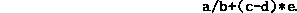Data Structures and Algorithms with Object-Oriented Design Patterns in C## Projects

1. Devise an algorithm to compute the height of a tree. Write an implementation of your algorithm as the Height method of the AbstractTree class introduced in Program.
2. Devise an algorithm to count the number of internal nodes in a tree. Write an implementation of your algorithm as the get accessor for the Count property of the AbstractTree class introduced in Program.
3. Devise an algorithm to count the number of leaves in a tree. Write an implementation of your algorithm as a method of the AbstractTree class introduced in Program.
4. Devise an abstract (generic) algorithm to compare trees. (See Exercise). Write an implementation of your algorithm as the CompareTo method of the AbstractTree class introduced in Program.
5. The Enumerator class introduced in Programdoes a preorder traversal of a tree.
1. Write an enumerator class that does a postorder traversal.
2. Write an enumerator class that does a breadth-first traversal.
3. Write an enumerator class that does an inorder traversal. (In this case, assume that the tree is a BinaryTree).
6.   Complete the GeneralTree class introduced in Programby providing suitable definitions for the following operations: IsEmpty, IsLeaf, Degree, and CompareTo. Write a test program and test your implementation.
7. Complete the NaryTree class introduced in Programby providing suitable definitions for the following operations: Purge, IsLeaf, Degree, and CompareTo. Write a test program and test your implementation.
8.   Complete the BinaryTree class introduced in Programby providing suitable definitions for the following operations: IsEmpty, IsLeaf, Degree, Key, AttachKey, DetachKey, AttachLeft, DetachLeft, AttachRight, DetachRight, and GetSubtree. Write a test program and test your implementation.
9. Write a visitor that draws a picture of a tree on the screen.
10. Design and implement an algorithm that constructs an expression tree from an infix expression such asHint: See Project.Copyright © 2001 by Bruno R. Preiss, P.Eng. All rights reserved.